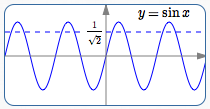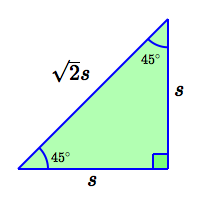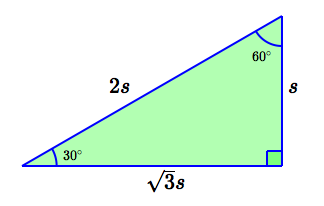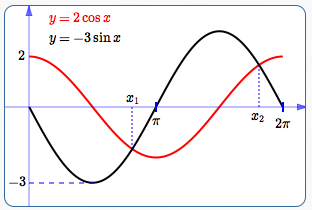﻿ Solving Simple Trigonometric Equations

# Solving Simple Trigonometric Equations

by Dr. Carol JVF Burns (website creator)
Follow along with the highlighted text while you listen!
• PRACTICE (online exercises and printable worksheets)

A trigonometric equation is an equation that uses at least one variable inside a trigonometric function.

Here are some examples of trigonometric equations. Follow the links to see the solutions.

• Example 1:   a single trigonometric function with input $\,x\,,$ no other appearance of the variable:
$\sqrt 2\sin x - 1 = 0$
• Example 2:   a single trigonometric function with input $\,kx\,,$ no other appearance of the variable:
$2\cos(3x) = 1$
$2\sin^2 x - \sin x - 1 = 0$
• Example 4:   use graphical methods to approximate solutions to trigonometric equations:
find all solutions of the equation ‘$\,2\cos x = -3\sin x\,$’ in the interval $\,[0,2\pi]\,$
• Example 5:   use WolframAlpha to solve trigonometric equations:
$\tan(5x-3)\sec(x^2 - 7) = 4$

Note that an equation like ‘$\,5 - x\sin 3 = 7\,$’ is not a trigonometric equation,
because it does not have a variable inside a trigonometric function.
Here, $\,\sin 3\,$ is just a constant. This is a linear equation in one variable with unique solution $\displaystyle\,x = \frac{-2}{\sin 3}\,.$

## Example 1:

### (a single trigonometric function with input $\,x\,,$ no other appearance of the variable)

The equation ‘$\,\sqrt 2\sin x - 1 = 0\,$’ is a trigonometric equation because of the ‘$\,x\,$’ inside the sine function.
Note that there is no other appearance of $\,x\,$ in this equation.
In cases such as this, we start by isolating the trigonometric function containing the variable.
(Recall that isolate means to get all by itself on one side of the equation.)

$\sqrt 2\sin x - 1 = 0$ original equation
$\displaystyle\sin x = \frac{1}{\sqrt 2}$ Isolate the trigonometric function with variable input:
add $\,1\,$ to both sides (using the addition property of equality);
divide both sides by $\,\sqrt 2\,$ (using the multiplication property of equality).

• Is the equation ever true? Can an output from the sine function ever equal $\displaystyle\,\frac{1}{\sqrt 2}\,$?
The range of the sine function is $\,[-1,1]\,.$
Since $\,\sqrt 2 \approx 1.4 > 1\,,$ we have $\,\frac{1}{\sqrt 2} \approx 0.7 < 1 \,.$
So, the number $\,\frac{1}{\sqrt 2}\,$ is in the range of the sine function.
Thus, there is at least one value for which the equation is true.
• How many solutions does the equation have?
A quick sketch (below) show that there are infinitely many solutions.
This is typical of trigonometric equations—they often have infinitely many solutions,
because of the periodic nature of trigonometric functions.reporting the solutions in degrees:

$x = 45^\circ + {360k\,}^\circ\,$ for all integers $\,k\,,$ or

$x = 135^\circ + {360k\,}^\circ\,$ for all integers $\,k\,$

$\displaystyle x = \frac{\pi}{4} + 2\pi k\,$ for all integers $\,k\,,$ or

$\displaystyle x = \frac{3\pi}{4} + 2\pi k\,$ for all integers $\,k\,$
Precisely what angles have sine equal to $\,\frac{1}{\sqrt 2}\,$?
From the special triangle below, we see that $\,\sin 45^\circ = \frac{1}{\sqrt 2}\,$;
so, $\,45^\circ\,$ (or $\,\displaystyle\frac{\pi}{4}\,$ radians) is one of the solutions of the equation.Recall that the sine function gives the $y$-values of points on the unit circle.
From the unit circle above, we can now get all the angles with $y$-value equal to $\,\frac{1}{\sqrt 2}\,,$ as follows:
Recall that, when laying off angles:
counterclockwise is the positive direction
and
clockwise is the negative direction

Also recall that the integers are: $$\cssId{s61}{\ldots, -3,-2,-1,0,1,2,3,\ldots}$$ Start at the terminal point for $\,45^\circ\,,$ in the first quadrant:
 Rotate counterclockwise (up) to get additional solutions: $45^\circ+ 360^\circ\,$ $45^\circ+ 2\cdot360^\circ\,$ $45^\circ+ 3\cdot360^\circ\,,$ and so on Rotate clockwise (down) to get additional solutions: $45^\circ - 360^\circ\,$ $45^\circ - 2\cdot360^\circ\,$ $45^\circ - 3\cdot360^\circ\,,$ and so on
See the pattern? Together, these give all the solutions in the first quadrant: $$\cssId{s73}{x = 45^\circ + {360k\,}^\circ\ \ \text{for all integers } k}$$ Next, start at the terminal point for $\,180^\circ - 45^\circ = 135^\circ\,,$ in the second quadrant:
 Rotate counterclockwise (down) to get additional solutions: $135^\circ+ 360^\circ\,$ $135^\circ+ 2\cdot360^\circ\,$ $135^\circ+ 3\cdot360^\circ\,,$ and so on Rotate clockwise (up) to get additional solutions: $135^\circ - 360^\circ\,$ $135^\circ - 2\cdot360^\circ\,$ $135^\circ - 3\cdot360^\circ\,,$ and so on
See the same pattern? Together, these give all the solutions in the second quadrant: $$\cssId{s85}{x = 135^\circ + {360k\,}^\circ\ \ \text{for all integers } k}$$

## Example 2:

### (a single trigonometric function with input $\,kx\,,$ no other appearance of the variable)

The equation ‘$\,2\cos(3x) = 1\,$’ is a trigonometric equation because of the ‘$\,x\,$’ inside the cosine function.
Note that there is no other appearance of $\,x\,$ in this equation.
Again, we start by isolating the trigonometric function containing the variable.

$\,2\cos(3x) = 1\,$ original equation
$\displaystyle\cos(3x) = \frac{1}{2}$ Isolate the trigonometric function with variable input.
The cosine function takes on the value $\,\frac 12\,$ infinitely many times,
so again there are infinitely many solutions.
to get $\,x\,$ in degrees:

$3x = 60^\circ + {360k\,}^\circ\,$ for all integers $\,k\,,$ or

$3x = -60^\circ + {360k\,}^\circ\,$ for all integers $\,k\,$

to get $\,x\,$ in radians:

$\displaystyle 3x = \frac{\pi}{3} + 2\pi k\,$ for all integers $\,k\,,$ or

$\displaystyle 3x = -\frac{\pi}{3} + 2\pi k\,$ for all integers $\,k\,$
For the moment, ignore the ‘$\,3x\,$’ inside the cosine function. (We'll use it in a minute.)
Ask:   What angles have cosine equal to $\displaystyle\,\frac{1}{2}\,$?
From the special triangle below, we see that $\displaystyle\,\cos 60^\circ = \frac{1}{2}\,.$Recall that the cosine function gives the $x$-values of points on the unit circle.
As in Example 1, get all the angles with $x$-value equal to $\,\frac{1}{2}\,$: $$\cssId{s109}{ 60^\circ + {360k\,}^\circ \ \ \text{ for all integers k } \quad \text{(in quadrant I)}} \tag{*}$$ $$\cssId{s110}{-60^\circ + {360k\,}^\circ \ \ \text{ for all integers k } \quad \text{(in quadrant IV)}} \tag{**}$$ These are all possible angles with cosine equal to $\,\frac 12\,.$

Now, we'll use the ‘$\,3x\,$’.
Note that if $\,x\,$ has units of degrees, then $\,3x\,$ also has units of degrees.
The angle inside the cosine function (which in this example is $\,3x\,$)
must equal one of the angles in (*) or (**), in order to have cosine equal to $\,\frac 12\,.$
Thus, for all integers $\,k\,,$ and for $\,x\,$ in degrees: $$\begin{gather} \cssId{s117}{3x = 60^\circ + {360k\,}^\circ}\cr \cssId{s118}{\text{or}}\cr \cssId{s119}{3x = -60^\circ + {360k\,}^\circ} \end{gather}$$
degree measure:
$x = 20^\circ + {120k\,}^\circ\,$ for all integers $\,k\,,$ or
$x = -20^\circ + {120k\,}^\circ\,$ for all integers $\,k\,$

$\displaystyle x = \frac{\pi}{9} + \frac{2\pi k}{3}\,$ for all integers $\,k\,,$ or
$\displaystyle x = -\frac{\pi}{9} + \frac{2\pi k}{3}\,$ for all integers $\,k\,$
Solve for $\,x\,.$
Done!

## Example 4:

### (use graphical methods to approximate solutions to trigonometric equations)

Graphical methods for solving equations (and inequalities) were studied thoroughly in earlier sections:

Even if you're working with a simple equation (as in Examples 1 and 2),
graphical methods are great for giving yourself confidence in your answers and/or catching mistakes.
Graphical methods typically provide approximate solutions, not exact solutions.

The equation ‘$\,2\cos x = -3\sin x\,$’ is a trigonometric equation because there is at least one variable inside a trigonometric function.
In this equation, there are two trigonometric functions with variable inputs;
isolating either one would still cause a variable expression (not a constant) to appear on the other side of the equation.
Thus, the technique illustrated in Example 1 and Example 2 doesn't work.

Both sides of the equation ‘$\,2\cos x = -3\sin x\,$’ are easy to graph—it is well-suited to graphical methods.
Graph the left-hand side; graph the right-hand side; approximate the intersection point(s) in a desired interval.

 $2\cos x = -3\sin x$ original equation; find solutions in the interval $\,[0,2\pi]\,$ $x\approx 2.6\,$   or   $\,x\approx 5.7\,$ Graph, on the interval $\,[0,2\pi]\,$: the left-hand side of the equation (in red): cosine curve, vertical stretch by a factor of $\,2\,$ the right-hand side of the equation (in black): sine curve, vertical stretch by a factor of $\,3\,,$ reflect about the $x$-axisIt is clear there are exactly two solutions in the interval $\,[0,2\pi]\,$; these correspond to the intersection points of the two graphs: $\,x_1\,$ is a bit less than $\,\pi\,$:   $\color{red}{2\cos x_1} \approx -3\sin x_1$ $\,x_2\,$ is a bit less than $\,2\pi\,$:   $\color{red}{2\cos x_2} \approx -3\sin x_2$ A graphing calculator or WolframAlpha (see Example 5) can be used to get decimal approximations for $\,x_1\,$ and $\,x_2\,.$

## Example 5:

### (use WolframAlpha to solve trigonometric equations)

WolframAlpha (opens in a new window) is a powerful, reliable, and free resource for mathematical information.
WolframAlpha understands conventional math syntax and abbreviations.
You can also type in a reasonable guess, and WolframAlpha will try to make sense of it.

For example, if you type in  ‘sine of x’  and press Enter, the  ‘Input:’  area will display ‘$\,\sin(x)\,$’.
This lets you know that WolframAlpha has understood you correctly.
Always check the  ‘Input:’  area, to make sure that you and WolframAlpha are on the same page!

Try solving each of the examples from this page (or the exercises) at WolframAlpha.
WolframAlpha is a good resource for checking answers, gaining additional insight, or when you just need a quick reliable answer.
You can cut-and-paste each of the following. Have fun!

tan(5x-3)sec(x^2 - 7) = 4

sin(x) = 1/sqrt(2)

2cos(3x) = 1

2sin^2 x - sin x - 1 = 0

2cos(x) = -3sin(x), 0 <= x <= 2pi
(WolframAlpha gives exact solutions; click ‘Approximate forms’ if desired)
Master the ideas from this section